×#### Thank you for registering.

One of our academic counsellors will contact you within 1 working day.

Click to Chat

1800-1023-196

+91-120-4616500

CART 0

• 0

MY CART (5)

Use Coupon: CART20 and get 20% off on all online Study Material

ITEM
DETAILS
MRP
DISCOUNT
FINAL PRICE
Total Price: Rs.

There are no items in this cart.
Continue Shopping```Indefinite Integral

Table of Content

What do we mean by an Indefinite Integral?

Methods of Integration

Integration by Parts

Integration by Substitution

Integration by Partial Fractions

Trigonometric Integrals

Related ResourcesIndefinite integral is an important component of integral calculus. It lays the groundwork for definite integral. Students are advised to practice as many problems as possible as only practice can help in achieving perfection in indefinite integrals.

Integration is used in dealing with two essentially different types of problems:

(1) The first type of problems are those in which the derivative of a function, or its rate of change, or the slope of its graph, is known and we want to find the function. We are therefore required to reverse the process of differentiation. This reverse process is known as anti-differentiation and it is often termed as finding of a primitive function or finding an indefinite integral.

(2) The second type leads to the definition of the definite integral. Definite integrals are used for finding area, volume, centre of gravity, moment of inertia, work done by a force and various such applications.

Remark:

This integral  is  called  an  indefinite  integral  because  its  value  is  not  fully determined until the end points are specified. This ambiguity is dealt with by the addition of the constant C at the end. This constant of integration is added to the end because there are, in fact, an infinite number of solutions to the integral.

The topic holds great importance from examination point of view and is widely used in modern sciences as well as in engineering entrance examinations. They account to many lengthy numericals and key derivations.

Various topics covered in this chapter include:

Topics Covered:

Basic Concepts in Indefinite Integral

Methods of Integration-Integration by Substitutiion

Standard Substitutions

Indirect Substitution

Integration by Parts

Integration by Partial Fractions

Algebraic Integrals

Trigonometric Integrals

Solved Examples of Indefinite Integral

Those willing to go into the intricacies of the topics are advised to refer the following sections. Here, we shall discuss all the topics in brief:

What do we mean by an indefinite integral?

Indefinite integration is also termed as antidifferentiation and as the name suggests, it is the reverse process of differentiation.

Suppose we have a function f and we intend to find a function F such that F’ = f, then in this case F is said to be the indefinite integral or the anti-derivative of the function f. Mathematically, we write it as,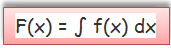where f(x) is called the integrand, x is called the integration variable and ‘c’ is the constant of integration.

In fact,iff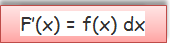Various basic and important formulae used in indefinite integrals have been discussed in the coming sections.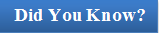Inverse property of indefinite integrals:

d/dx ∫ f(x) dx = f(x)

∫ f’(x) dx = f(x) + c

Two antiderivatives of a given function can only differ by a constant, i.e. if F is an antiderivative of a continuous function f, then any other antiderivative G of the function f is of the form G(x) = F(x) + c

If ∫ f(x) dx = g(x) + c, then ∫ f(ax + b) dx = 1/a g(ax + b) + c, where a and b are constants such that a ≠ 0.

Constant is an important component in indefinite integration.

Methods of Integration

Generally speaking, there are three methods of integration used for solving indefinite integrals which are: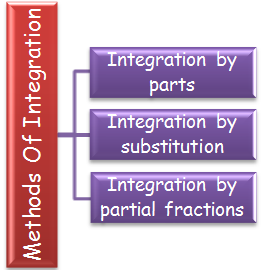At times, questions on integration can be solved directly by application of direct formulae. But, usually the questions asked in competitive examinations are not very simple and can’t be solved by direct application of formulae.

If the integrand is not a derivative of a known function, then the corresponding integrals cannot be found directly. In order to find the integrals of complex problems, generally three rules of integration are used.

(1) Integration by parts.

(2) ?Integration by substitution or by change of the independent variable.

(3) Integration by partial fractions.

We shall only give a brief outline of these methods as they have been discussed in detail in the coming sections:

Integration by Parts

If u and v be two functions of x, then the integral of product of these two functions is given by: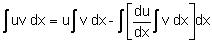Note: In applying the above rule care has to be taken in the selection of the first function (u) and the second function (v) depending on which function can be integrated easily. Normally we use the following methods for making this choice:

If both of the functions are directly integrable then the first function is chosen in such a way that the derivative of the function thus obtained under integral sign is easily integrable. Usually we use the following preference order for the first function.

(Inverse, Logarithmic, Algebraic, Trigonometric, Exponent)

This rule is called as ILATE which states that the inverse function should be assumed as the first function while performing integration. Hence, the functions are to be assumed in the order from left to right depending on the type of functions involved. Various other cases have been discussed in detail in the later sections.

Example

Evaluate ∫ ex (1/x – 1/x2)dx.

Solution:

Let I = ∫ ex (1/x – 1/x2)dx

Here f(x) = 1/x, f'(x) = –1/x2

Hence I = ex / x + c

Integration by Substitution

?It is not always possible to find the integrals of complicated functions by observation and hence we need methods like substitution for solving them. The substitution method has the following parts:

?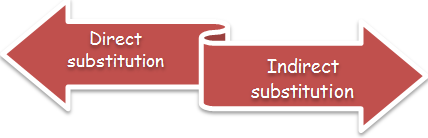Besides direct and indirect methods, sometimes questions can also be solved by some standard substitutions. The direct and indirect substitutions have been discussed in detail in the section on substitution method. We list here some of the standard substitutions.

Some Standard Substitutions

For terms of the form x2 + a2 or √x2 + a2, put x = a tan θ or a cot θ

For terms of the form x2 - a2 or √x2 – a2 , put x = a sec θ or a cosec θ

For terms of the form a2 - x2 or √x2 + a2, put x = a sin θ or a cos θ

If both √a + x, √a – x,  are present, then put x = a cos θ.

For the type √(x–a)(b–x), put x = a cos2θ + b sin2θ

For the type (√x2 + a2 ± x)n or (x ± √x2 – a2)n, put the expression within the bracket = t.

For 1/(x+a)n1 (x+b)n2, n1,n2 ∈ N (and > 1), again put (x + a) = t(x + b)

Example:

Evaluate ∫ x3 tan–1 x4/(1 + x8) dx.

Solution:

Put tan–1 x4 = t

Then ⇒ 4x3/(1 + x8) dx = dt

Hence, using this in the above integral we have

I = 1/4  ∫ t dt

= 1/4 t2/2 + A

= 1/8 (tan–1 x4)2 + A.

Integration by Partial Fractions

If P(x) and Q(x) are two polynomials, then P(x)/Q(x) is called a rational function. It may further be classified as a proper rational function or an improper rational function.

If deg P(x) < deg Q(x), then it is called a proper rational function.

If deg P(x) ≥ deg Q(x), then it is called an improper rational function.

If P(x)/Q(x) is an improper rational function then we divide P(x) by Q(x) so as to express the rational function P(x)/Q(x) in the form a(x) + b(x)/Q(x), where a(x) and b(x) are polynomials such that degree of b(x) is less than that of Q(x).

In order to write P(x)/Q(x) in partial fractions, first of all we write

Q(x) = (x – a)k ... (x2 + αx + β)r ... where binomials are different, and then setwhere A1, A2, ..., Ak, M1, M2, ......, Mr, N1, N2, ...... ,Nr are real constants to be determined. These are determined by reducing both sides of the above identity to integral form and equating the coefficients of equal powers of x, which gives a system of linear equations in the coefficient. (This method is called the method of comparison of coefficients). The constants can also be obtained by substituting suitably chosen numerical values of x in both sides of the identity.

Example:

Evaluate ∫ 1/sin x (2 + cos x – 2 sinx) dx.

Solution:

Put tan x = t ⇒ sec2.x/2 . 1/2 dx = dt

⇒ dx = 2/sec2 x/2 dt

⇒ 2/1 + t2  dt, then we haveThis expands into simple fractions

(1 + t2)/ t(t – 3) (t -1) = A/t + B/(t–3) + C/(t -1)

After solve the coefficients, A = 1/3; B = 5/3; C = –1

Hence I = 1/3 ∫ dt / t + 5/3 ∫ dt/ (t -3) – ∫ dt / (t-1)

= 1/3 In |t| + 5/3 |t-3| - In |t-1| + c

= 1/3 In |tan x/2| + 5/3 |tan x/2 –3| – ln | tan x/2 – 1 | + c.

Trigonometric Integrals

Integrals of the form ∫ R ( sinx, cosx) dx:

Here R is a rational function of sin x and cos x. This can be translated into integrals of a rational function by the substitution: tan(x/2) = t. This is the so called universal substitution. In this case

sin x = 2t/(1+t2) ; cos x = (1-t2)/(1+t2), x = 2 tan-1 t; dx = 2 dt/(1 + t2)

Sometimes, instead of the substitution tan x/2 = t, it is more advantageous to make the substitution cot x/2 = t

Universal substitution often leads to very cumbersome calculations. Indicated below are those cases where the aim can be achieved with the aid of simpler substitutions.

(a) If R (-sin x, cos x) = -R(sin x, cos x), substitute cos x = t

(b) If R (sin x, -cos x) = -R(sin x, cos x), substitute sin x = t

(c) If R (-sin x, - cos x) = R(sin x, cos x), substitute tan x = t

Various other cases of substitution in case of trigonometric functions have been discussed in detail in the later sections.

Example:

Evaluate ∫ √sin x cos x dx.

Solution:

Put sin x = t

Then cos dx  = dt

Substituting these values in the given question we have,

∫ t1/2 = 2/3 t3/2 + c = 2/3 (sin x)3/2 + c

Some Basic Properties of Indefinite Integral

∫ k f(x) dx = k ∫ f(x) dx, where k is any number. Hence, a constant can be taken out of the integral sign.

∫ - f(x) dx = - ∫ f(x), this actually follows from the above property only for k = -1.

∫ (f(x) ± g(x)) dx = ∫ f(x) dx ±  ∫ g(x)) dx, this property can be extended to any number of functions.

The same cannot be said for multiplication and division of functions i.e.

.∫ f(x) / g(x) dx ≠ ∫ f(x) dx / ∫ g(x) dx and similarly

∫ f(x) . g(x) dx ≠ ∫ f(x) dx . ∫ g(x)) dx?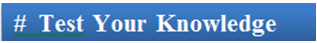Q1. We need to integrate an inverse and a logarithmic function. Then the order of functions should be:

(a) inverse function as the first function and logarithmic as the second.

(b) the functions can be chosen in any order.

(c) logarithmic function should be chosen as the first and inverse as the second.

(d) can’t say

Q2. Indefinite integration is also termed as

(a) irregular integration

(b) anti differentiation

(c) none of these

(d) reverse integration

Q3. While using integration by parts, the sequence of the functions is decided by the rule

(a) TIALE

(b) IATE

(c) ILETA

(d) ILATE

Q4. f(x)/g(x) is said to be a proper rational function if

(a) deg f(x) > deg g(x)

(b) deg f(x) = deg g(x)

(c) deg f(x) < deg g(x)

(d) deg f(x) ≠ deg g(x)

Q5. Two integrals of the same function

(a) are exactly the same

(b) are entirely different

(c) differ only by a constant

(d) may or may not be same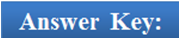Q5

Q5

Q5

Q5

Q5

(a)

(b)

(d)

(c)

(c)

Related Resources

For getting an idea of the type of questions asked, refer the previous year papers.?

You may wish to refer definite integral.

To read more, Buy study materials of Indefinite integral comprising study notes, revision notes, video lectures, previous year solved questions etc. Also browse for more study materials on Mathematics here.

```### Course Features

• 731 Video Lectures
• Revision Notes
• Previous Year Papers
• Mind Map
• Study Planner
• NCERT Solutions
• Discussion Forum
• Test paper with Video Solution